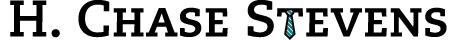Posts with tag "lambda":

More Stupid Higher-Order Function Tricks

posted on: Thursday, March 15, 2012 (11:23 am) by Chase Stevens

A while ago, a friend of mine who was unfamiliar with python asked me how to go about parsing a CSV into a list of lists. Moreover, the data included integers which needed to be parsed as such (and not as strings). My solution was as follows:

(lambda f: [map((lambda x: x.isdigit() and int(x) or x),(line.split(','))) for line in f.readlines()]) #takes a file
Just yesterday, another friend of mine had accidentally merged and subsequently sorted two excel spreadsheets, and overwrote one with the result. She asked if I could take the merged file and the remaining original file and re-construct the file she had overwritten. Incorporating the CSV parsing function I had written earlier, I wrote this:
full = (lambda f: [map((lambda x: x.isdigit() and int(x) or x),(line.split(','))) for line in f.readlines()])(askopenfile())
exclude = (lambda f: [map((lambda x: x.isdigit() and int(x) or x),(line.split(','))) for line in f.readlines()])(askopenfile())
(lambda f: map(f.write,(map(','.join,filter((lambda x: x not in exclude),full)))))(asksaveasfile())

Tags: code, csv, filter, lambda, map, programming, python, tkinter

Plotting Simple Functions in 3 Lines with Python

posted on: Tuesday, March 6, 2012 (11:13 am) by Chase Stevens

You can hardly even tell that I took a course on functional programming last semester.

import turtle
eq = "(x ** 2) / 1.5"
map((lambda x: turtle.setx(x) or turtle.sety(x)),[(n,(eval(eq.replace("x","(" + str(n) + ")")))) for n in range(0,100)])

Tags: code, lambda, math, programming, python